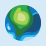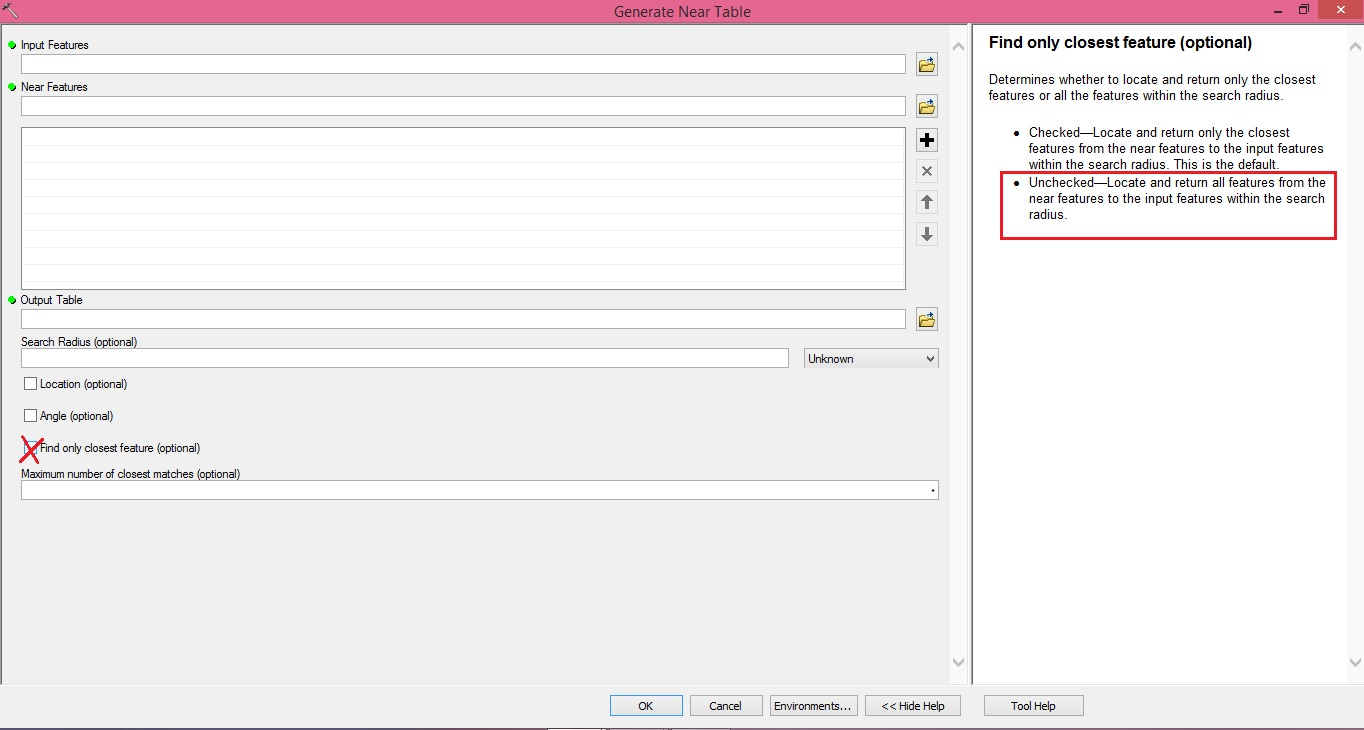# distance matrix between points

2227
7
04-12-2017 06:25 AMNew Contributor III

Hello all!

I cannot find the way how to create a distance matrix from a point layer. If I use the point distance tool from the proximity toolbox I just get a table with the distances between each point within the layer but I need a 1:1 data matrix (rows and columns as points, values as distances and the diagonal with 0 values). There must be certainly a way how to create this…

I need the distance matrix for other calculations.

I am using ArcGIS 10.3.1 for Desktop (Advanced Licence).

Do you have any ideas?

Thanks for four support.

Tags (4)
7 RepliesbyMVP Esteemed Contributor

I have posted in my blog a number of times on distance calculations.  You can use scipy.distance.cdist to produce a squareform matrix, however, I prefer to use a pure numpy based solution using einsum which does the same trick.

Here is the def from a larger module I maintain.

``````def e_dist(a, b, metric='euclidean'):
"""Distance calculation for 1D, 2D and 3D points using einsum
: a, b   - list, tuple, array in 1,2 or 3D form
: metric - euclidean ('e','eu'...), sqeuclidean ('s','sq'...),
:-----------------------------------------------------------------------
"""
a = np.asarray(a)
b = np.atleast_2d(b)
a_dim = a.ndim
b_dim = b.ndim
if a_dim == 1:
a = a.reshape(1, 1, a.shape)
if a_dim >= 2:
a = a.reshape(np.prod(a.shape[:-1]), 1, a.shape[-1])
if b_dim > 2:
b = b.reshape(np.prod(b.shape[:-1]), b.shape[-1])
diff = a - b
dist_arr = np.einsum('ijk,ijk->ij', diff, diff)
if metric[:1] == 'e':
dist_arr = np.sqrt(dist_arr)
dist_arr = np.squeeze(dist_arr)
return dist_arr‍‍‍‍‍‍‍‍‍‍‍‍‍‍‍‍‍‍‍‍‍‍``````

and here is simple set of data points so you can see how it works

``````a = np.arange(10).reshape(5,2)
a
array([[0, 1],
[2, 3],
[4, 5],
[6, 7],
[8, 9]])

e_dist(a,a)

array([[ 0.000,  2.828,  5.657,  8.485,  11.314],
[ 2.828,  0.000,  2.828,  5.657,  8.485],
[ 5.657,  2.828,  0.000,  2.828,  5.657],
[ 8.485,  5.657,  2.828,  0.000,  2.828],
[ 11.314,  8.485,  5.657,  2.828,  0.000]])‍‍‍‍‍‍‍‍‍‍‍‍‍‍‍``````

give it a shot.  3D distance can also be determine using einsum notation

You can also use an upper of lower matrix format if you want to make it easier to read

``````np.triu(e_dist(a, a))

array([[ 0.000,  2.828,  5.657,  8.485,  11.314],
[ 0.000,  0.000,  2.828,  5.657,  8.485],
[ 0.000,  0.000,  0.000,  2.828,  5.657],
[ 0.000,  0.000,  0.000,  0.000,  2.828],
[ 0.000,  0.000,  0.000,  0.000,  0.000]])‍‍‍‍‍‍‍``````New Contributor III

Hello Dan,

I just thought after using the “Point Distance” tool there will be another tool where I can simply convert the table with the distances into a data matrix.byMVP Esteemed Contributor

Not with your requirements, you might be able to cobble an OD matrix from excel but everything else in Arc* is a linear table formatRegular Contributor II

Did you try Generate Near Table tool without check Find only closest feature?byMVP Esteemed Contributor

except it doesn't return a matrix with 0's on the diagonalRegular Contributor II

Yes, You are right ,DanNew Contributor III

Hi Abdullah,

yeah I have already tried that but the result is the same as by using the “Point distance” tool with the only difference that there is a new column in the attribute table with the ranks of the distances but it remains a table…So I have to use the solution from Dan.